Function Repository Resource:

# ConchoidCurve

Compute a conchoid curve

Contributed by: Jan Mangaldan
 ResourceFunction["ConchoidCurve"][c,p,d] computes one branch of the conchoid of the curve c with respect to the fixed point p and distance d. ResourceFunction["ConchoidCurve"][c,p,d,-1] computes the other branch of the conchoid.

## Details

The conchoid of a curve with respect to a fixed point is the locus of points on a variable line passing through the fixed point and intersecting the curve, such that their distance from the intersection point is equal to a given distance d.

## Examples

### Basic Examples (2)

Conchoid of a circle:

 In:=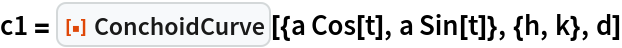Out=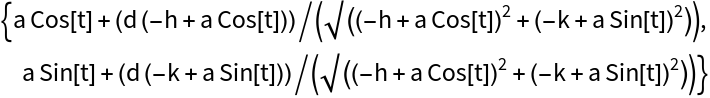Plot one branch of the conchoid:

 In:=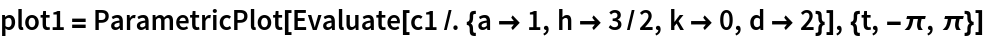Out=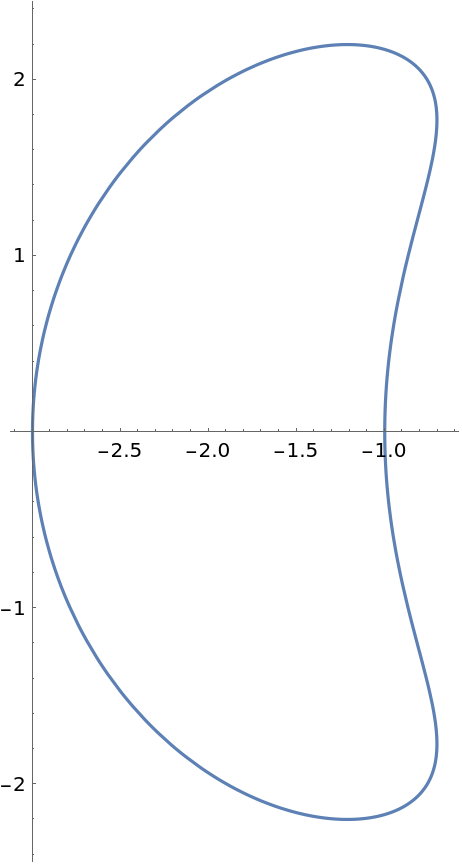Plot the other branch:

 In:=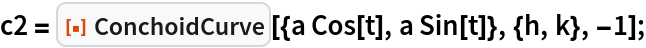In:=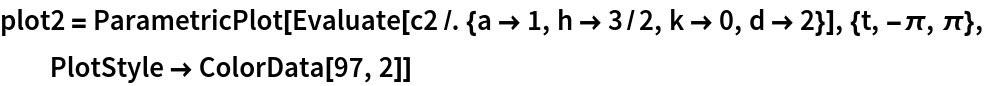Out=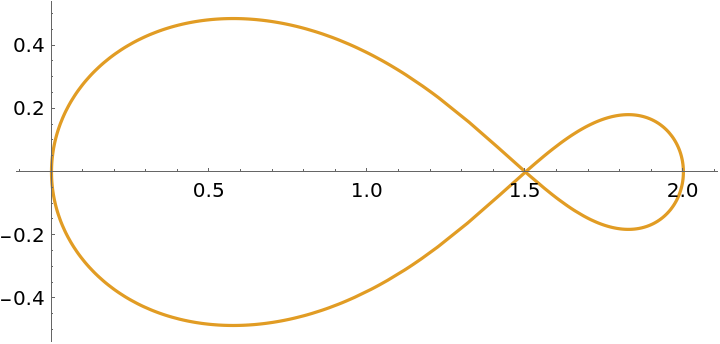Show both branches together:

 In:=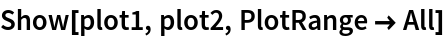Out=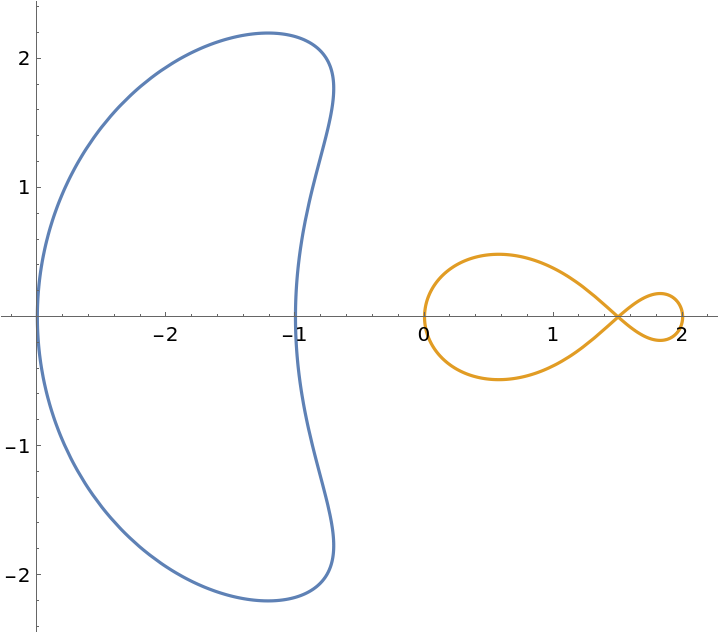Find the implicit Cartesian equation of the conchoid:

 In:=Out=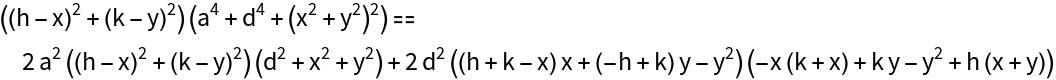The conchoid of Nicomedes is the conchoid of a line with respect to the origin:

 In:=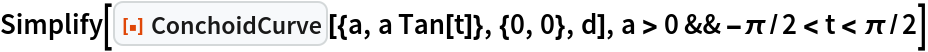Out=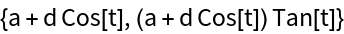In:=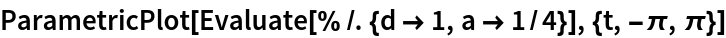Out=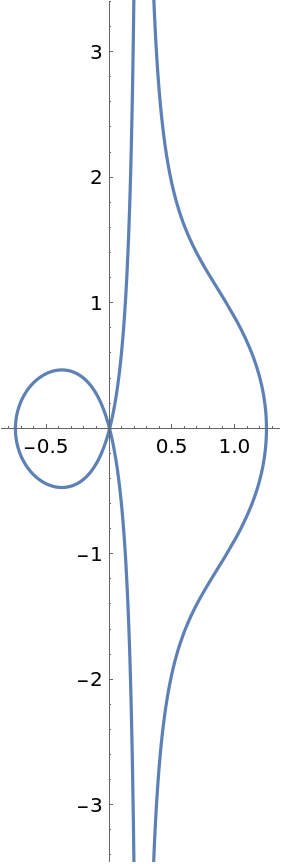## Version History

• 1.0.0 – 09 March 2021